cancel
Showing results for
Did you mean:

Fabric is Generally Available. Browse Fabric Presentations. Work towards your Fabric certification with the Cloud Skills Challenge.Helper II

## How to calculate bank balance per week

Hey guys,

I've been struggeling with this for a while now. I want to calculate the bank balance (composed of 3 bank accounts) per week.

Right now i tried calculating the sum, but that doesn't work; there is a closing value per day in the data, so if i sum it up, it will sum up all the closing values. I only need the total of the 3 accounts for the latest date.This is what i've tried. The first two parts in calculating the debitors en creditors work. But i need some help on the final part of calculating the bank balance.

1 ACCEPTED SOLUTION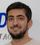Super User

In your Final Table create a Calculated Column:

``WeekDay=WEEKDAY('Final'[Datum])``

Then Create the measure below:

``````BankBalance =
SUMX (
FILTER (
'Final',
'Final'[WeekDay] = 7
|| 'Final'[Datum] = CALCULATE ( MAX ( Final[Datum] ), ALL ( Final ) )
),
'Final'[Saldo eind]
)``````

If I understand correctly, It is what you need. To work as expected, you need to put Week Column in Matrix as well. Then it would show the balance per week. If you want **bleep** in another format of visualization, let me know.

18 REPLIES 18Helper II

I have created this measure, and it displays the correct balance for the current week.

Now, how do i transform this into the IF statement to show it for each week?Somehow if i place it into the IF statement like this: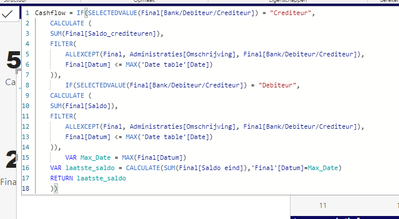The 'bank' row just dissapears from the matrix.Super UserSuper User

In your Final Table create a Calculated Column:

``WeekDay=WEEKDAY('Final'[Datum])``

Then Create the measure below:

``````BankBalance =
SUMX (
FILTER (
'Final',
'Final'[WeekDay] = 7
|| 'Final'[Datum] = CALCULATE ( MAX ( Final[Datum] ), ALL ( Final ) )
),
'Final'[Saldo eind]
)``````

If I understand correctly, It is what you need. To work as expected, you need to put Week Column in Matrix as well. Then it would show the balance per week. If you want **bleep** in another format of visualization, let me know.Helper II

Hi,

It now displays the same amout in every column.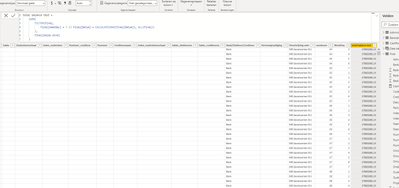Super User

It is not shown per week because you have not put the week column in the visual to separate.

I don't understand exactly how do you want to visualize it. But if you want it per week, you should put the week column in your matrix as well.Helper II

My bad, i had put in the week of another table. Now the bank balance is correct. However, it is currently week 13 and my table is displaying week 14 (so its one month ahead). How do i change this in power query?

Also after week 19 it jumps back to week 2. (and after week 29 it jumps back to 3)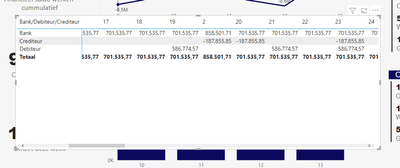Super User

You're Welcome @QwertyMartijn ,

I do not know your columns for debitor and creditor very well, but I think you need to calculate debitor and creditor totals weekly.
So you need to change your first 2 parts as below:

``````CALCULATE (
SUM ( Final[Saldo_Crediteuren] ),
FILTER ( FINAL, FINAL[WeekNo] = WEEKNUM ( MAX ( 'Date Table'[Date] ) ) )
)``````Super User

Instead of power query it is easier if you go to your date table and create a calculated column:

``WeekNo = WEEKNUM('Final'[Datum])``

Then put this column in your Matrix visual. Does it fix your problem?Helper II

That did fix it!

I only have one last thing. Can't thank you enough already, been working on this for way too long.

I pasted the part for the bank balance in my original cashflow measure.However, the creditors en debitors do not variate per week: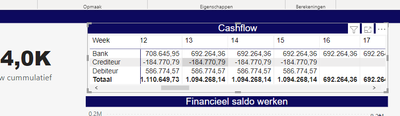Super User

Create it as a measure

Not a calculated column.

If you put the measure and Week in a table visual, it should work.Helper II

Somehow it wont slice on week. Maybe this total over all the weeks is correct, but it doesnt show up per week.Helper II

@MohammadLoran25 Thanks for all help already. But i accidentally clicked on 'accept as solution'.

Sorry if i was unclear. It should be sliced by week, as shown in the picture i posted. The measure that you suggested now sums up all the values of the 7th day of the week (which i think might be correct), but it is not sliced by week yet.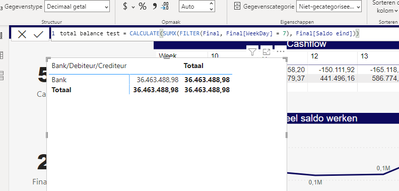Super User

I made an update in my message.

What do you mean by sliced per week?

You need to put the week column in your matrix as well. How do you want to show it per week?Super User

Okay

But how do you want it to be shown per week? By date slicer?

Or putting Week in the matrix?

Thanks.Super User

Try this:

TotalBalance =
CALCULATE (
SUM ( Final[Saldo eind] ),
FILTER ( ALL ( Final[Datum] ), Final[Datum] <= MAX ( 'Date Table'[Date] ) )
)

If this answer solves your problem, please give it a thumbs up and mark it as an accepted solution so the others would find what they need easier.

Regards,
LoranHelper II

Thanks for your reply. Somehow my 'bank' does not show up in the table anymore.Super User

Would you please share a sample data with desired result as well?Helper II

This is what the data looks like: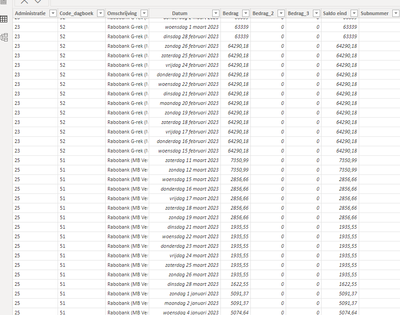There are multiple bank accounts (Omschrijving) and each have a starting amount (bedrag) and a closing amount for that date (saldo eind). The goal is to calculate the total of the different bank accounts for each week.

In my original code, the filters i used do filter per week, but the function SUM is wrong, because it just sums up al the closing amounts.Announcements#### Power BI Monthly Update - November 2023

Check out the November 2023 Power BI update to learn about new features.#### Fabric Community News unified experience

Read the latest Fabric Community announcements, including updates on Power BI, Synapse, Data Factory and Data Activator.#### The largest Power BI and Fabric virtual conference

130+ sessions, 130+ speakers, Product managers, MVPs, and experts. All about Power BI and Fabric. Attend online or watch the recordings.Top Solution Authors
Top Kudoed Authors
Users online (4,262)